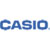# Casio® FX-300MSPLUS2 Scientific Calculator, 12-Digit LCD# Casio® FX-300MSPLUS2 Scientific Calculator, 12-Digit LCD

#### Item #CSOFX300MSPLUS2

• 229 built-in functions.
• Permitted on college entrance exams, such as SATs.
• Solar Plus protects memory.
• Auto power down feature.\$24.71/EA

229 built-in functions. Permitted on college entrance exams, such as SATs. Solar Plus means calculator has battery backup in dim light. Auto power down feature.

Power Source(s): Battery; Solar; Display Notation: Numeric; Number of Display Digits: 12; Display Characters x Display Lines: 12 x 2.

• Global Product Type Scientific Calculators
• Power Source(s) Battery; Solar
• Display Notation Numeric
• Number of Display Digits 12
• Display Characters x Display Lines 12 x 2
• Calculators Memory 10 Registers
• Calculators Display Type LCD
• Case Slide-On Hard Case
• Display Characters Height 4 mm
• Percent Key(s) No
• Fraction Calculations Yes
• Fraction/Decimal Conversions Yes
• Decimal Function Yes
• +/- Switch Key No
• Currency Exchange Function No
• Metric Conversion No
• Backspace Key Yes
• Double Zero Key No
• Amortization No
• Base Number Calculations No
• Bond Calculations No
• Cash Flow Calculations No
• Complex Number Calculations No
• Confidence Interval Calculating No
• Cost/Sell/Margin No
• Date Calculations No
• Depreciation Calculations No
• Entry Logic V.P.A.M.
• Equation Editor Yes
• Grand Total Key No
• Higher Mathematical Functions Algebra I & II; Biology; Geometry; Pre-Algebra; Sciences; Statistics; Trigonometry
• Hyperbolic Functions Yes
• Hypothesis Testing Yes
• Interest Rate Conversion No
• Item Count Function No
• Linear Regression Yes
• Loan Calculation No
• Logical (Boolean) Operations No
• Markup/Down Key No
• Matrices No
• Polar-Rectangular Conversion No
• Probability (Random Number) Yes
• Simultaneous Equations No
• Square Root Key Yes
• Tax Calculation No
• Time-Value-of-Money No
• Time/Date No
• Trig/Log Functions Yes
• Variable Regression No
• Size 3.03 x 6.36
• I/O Port No
• Replacement Batteries LR44
• Wall-Mountable No